# Keras入门（四）之利用CNN模型轻松破解网站验证码

2019/03/24 15:35

### 项目简介

在之前的文章keras入门（三）搭建CNN模型破解网站验证码中，笔者介绍介绍了如何用Keras来搭建CNN模型来破解网站的验证码，其中验证码含有字母和数字。   让我们一起回顾一下那篇文章的处理思路：

• 利用OpenCV对图像进行单个字符的切割，大概400多张图片；
• 对切割好的单个字符进行人工手动标记；
• 搭建合适的CNN模型，对标记好的数据集进行训练；
• 对于新的验证码，先切割单个字符，再对单个字符进行预测，组成总的预测结果。

这一次，笔者将会换种思路，使用CNN模型来破解网站的验证码。我们的数据集如下：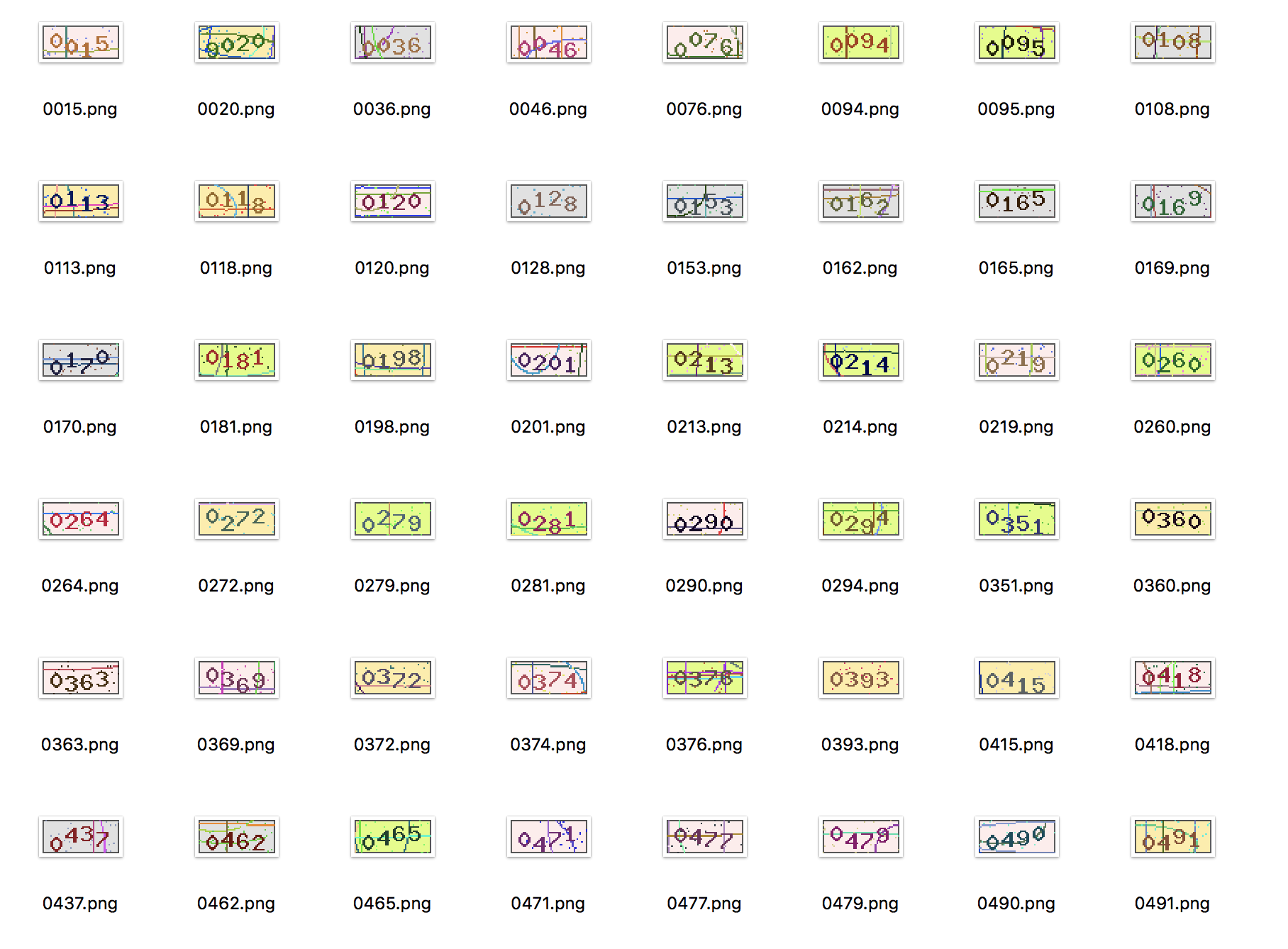• 直接对验证码进行标记，标记的结果见上图；
• 搭建合适的CNN模型对标记好的数据集进行训练；
• 对新验证码进行预测。

### 数据标记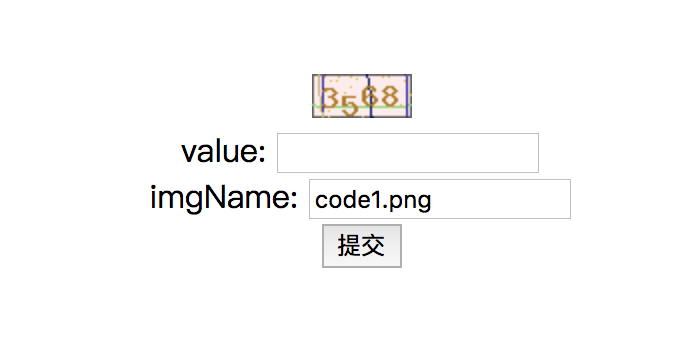### 模型训练

标记完验证码后，我们就利用这946张验证码作为训练数据，训练CNN模型。我们使用Keras框架，CNN模型的结构图如下：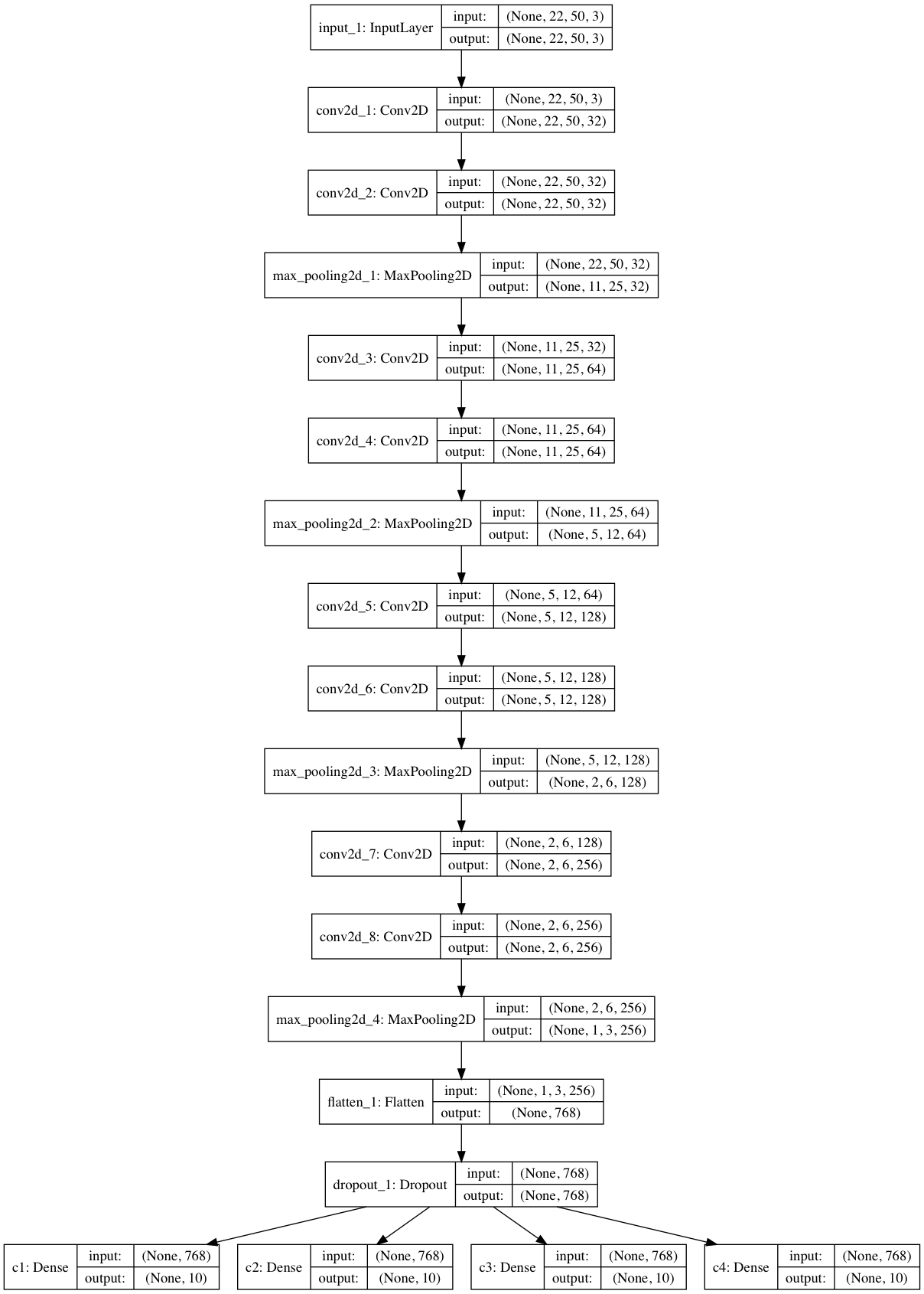# CNN模型训练

# -*- coding: utf-8 -*-
import cv2
import os
import random
import numpy as np
from keras.models import *
from keras.layers import *
from keras import callbacks

characters = '0123456789'
width, height, n_len, n_class = 50, 22, 4, 10

# 产生训练的一批图片，默认是32张图片
def gen(dir, batch_size=32):
X = np.zeros((batch_size, height, width, 3), dtype=np.uint8)
y = [np.zeros((batch_size, n_class), dtype=np.uint8) for _ in range(n_len)]
files = os.listdir(dir)
while True:
for i in range(batch_size):
path = random.choice(files)
filename = path[:4]
X[i] = imagePixel
for j, ch in enumerate(filename):
y[j][i, :] = 0
y[j][i, characters.find(ch)] = 1

yield X, y

input_tensor = Input((height, width, 3))
x = input_tensor

# 产生有四个block的卷积神经网络
for i in range(4):
# 卷积层
x = Conv2D(32 * 2 ** i, (3, 3), activation='relu', padding='same')(x)
x = Conv2D(32 * 2 ** i, (3, 3), activation='relu', padding='same')(x)
# 池化层
x = MaxPooling2D((2, 2))(x)

x = Flatten()(x)
x = Dropout(0.25)(x)

# 多输出模型，使用了4个'softmax'來分别预测4個字母的输出
x = [Dense(n_class, activation='softmax', name='c%d' % (i + 1))(x) for i in range(4)]
model = Model(inputs=input_tensor, outputs=x)
model.summary()

# 保存模型结构图
from keras.utils.vis_utils import plot_model
plot_model(model, to_file="./model.png", show_shapes=True)

model.compile(loss='categorical_crossentropy',
metrics=['accuracy'])

# 保存效果最好的模型
cbks = [callbacks.ModelCheckpoint("best_model.h5", save_best_only=True)]

dir = './result'
history = model.fit_generator(gen(dir, batch_size=8),      # 每次生成器会产生8张小批量的图片
steps_per_epoch=120,    # 每次的epoch要训练120批图片
epochs=50,                # 总共训练50次
callbacks=cbks,          # 保存最好的模型
validation_data=gen(dir),   # 验证数据也是用生成器來产生
validation_steps=10      # 用10组图片来进行验证
)

# 绘制损失值图像
import matplotlib.pyplot as plt

def plot_train_history(history, train_metrics, val_metrics):
plt.plot(history.history.get(train_metrics), '-o')
plt.plot(history.history.get(val_metrics), '-o')
plt.ylabel(train_metrics)
plt.xlabel('Epochs')
plt.legend(['train', 'validation'])

# 打印整体的loss与val_loss，并保存图片
plot_train_history(history, 'loss', 'val_loss')
plt.savefig('./all_loss.png')

plt.figure(figsize=(12, 4))

# 第一个数字的正确率
plt.subplot(2, 2, 1)
plot_train_history(history, 'c1_acc', 'val_c1_acc')

# 第二个数字的正确率
plt.subplot(2, 2, 2)
plot_train_history(history, 'c2_acc', 'val_c2_acc')

# 第三個数字的正確率
plt.subplot(2, 2, 3)
plot_train_history(history, 'c3_acc', 'val_c3_acc')

# 第四個数字的正确率
plt.subplot(2, 2, 4)
plot_train_history(history, 'c4_acc', 'val_c4_acc')

# 保存图片
plt.savefig('./train.png')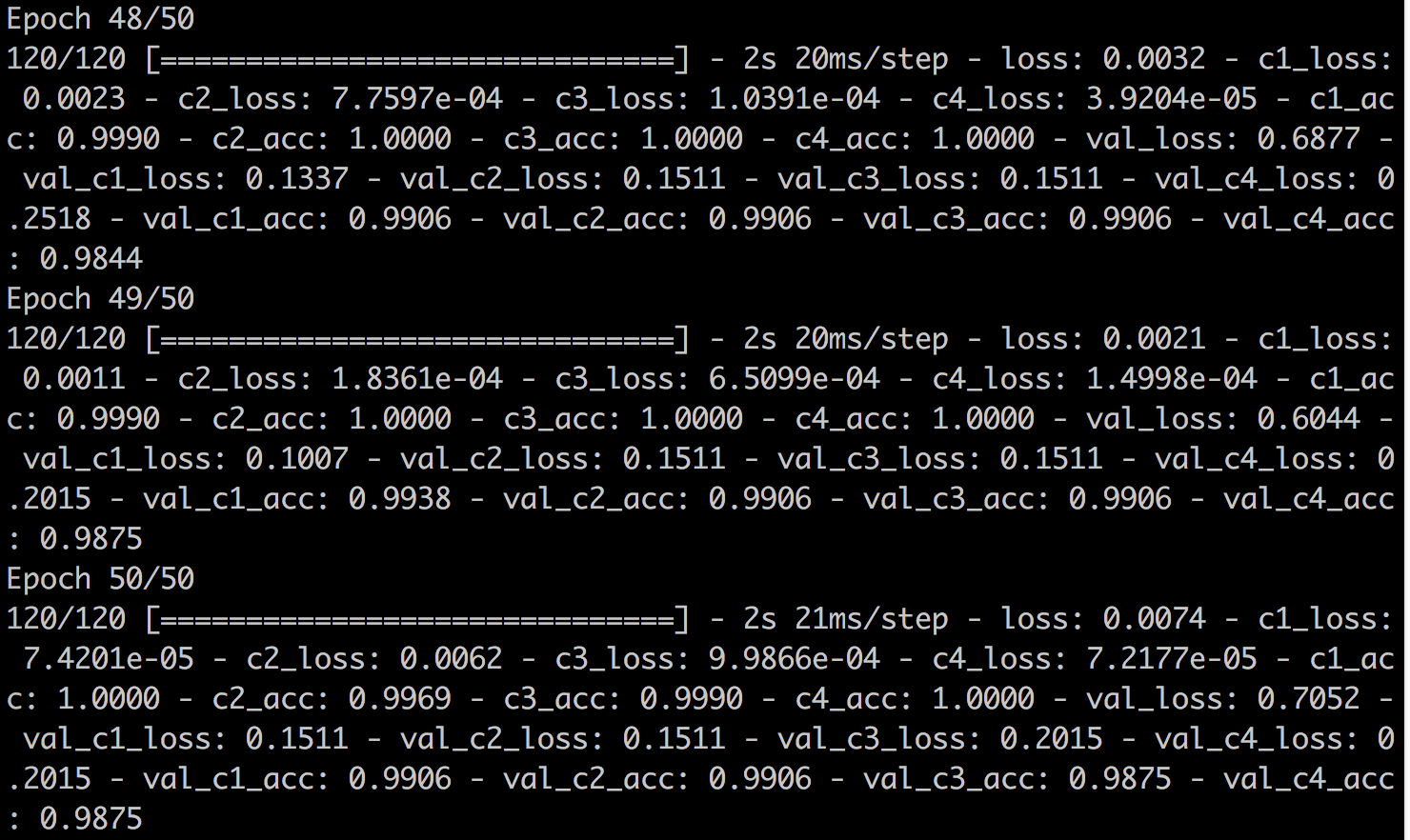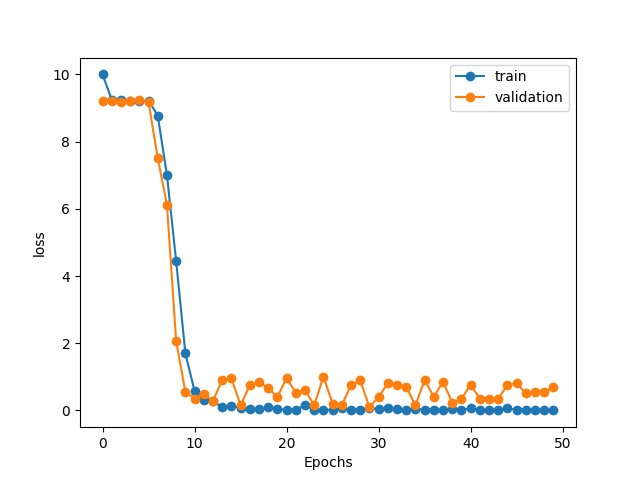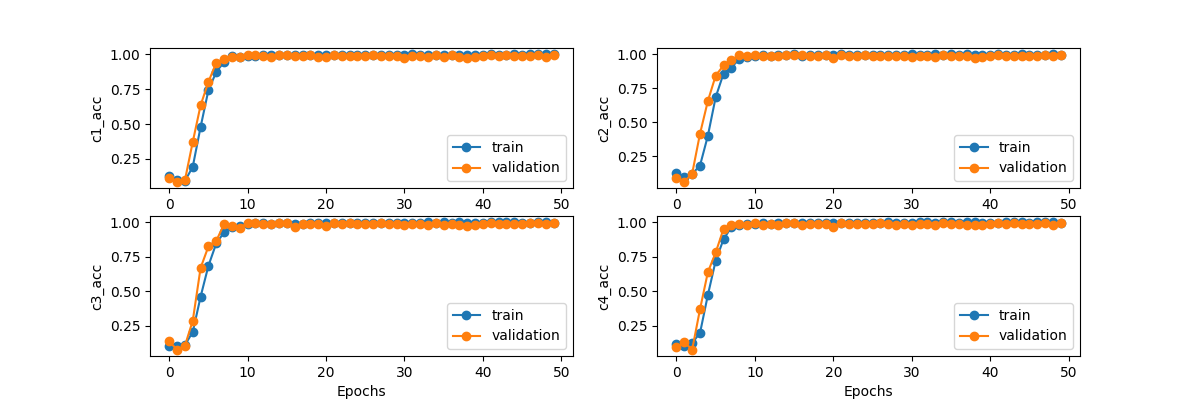### 模型预测

新的验证码共有20张，如下：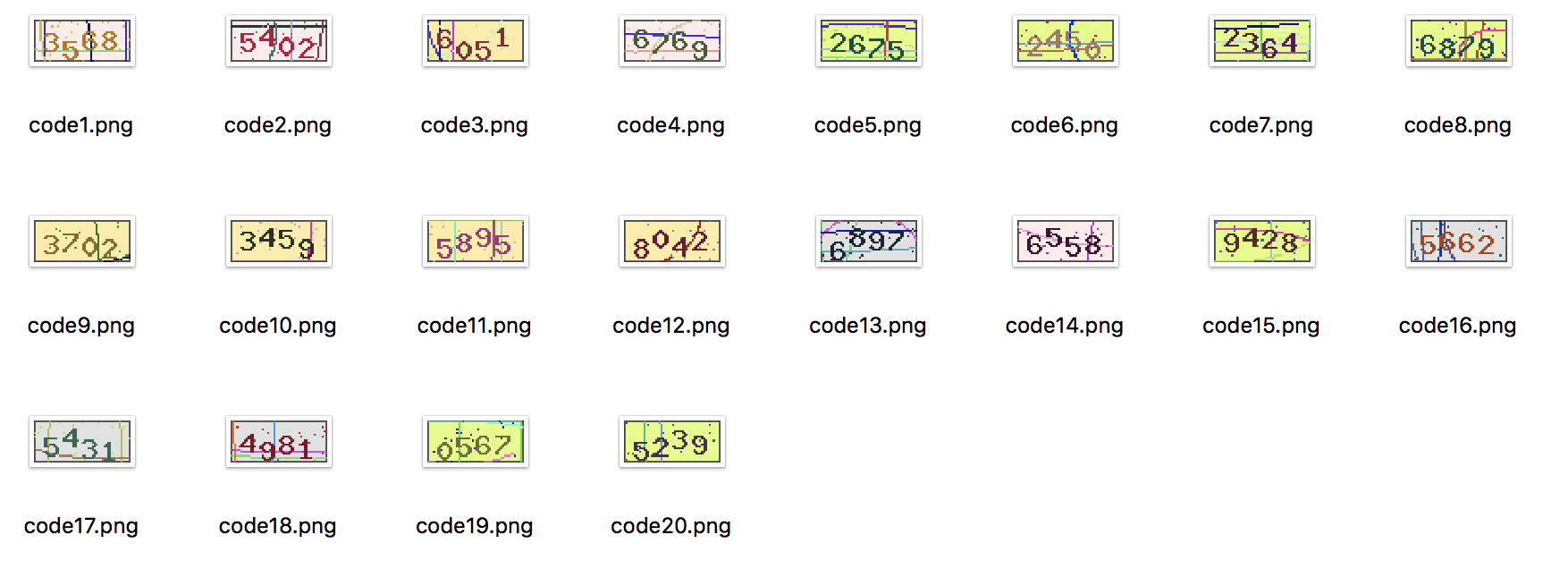# 使用训练好的CNN模型对新图片进行预测

# -*- coding: utf-8 -*-
import cv2
import numpy as np

# 导入训练好的模型

batch_size = 20
width, height, n_len, n_class = 50, 22, 4, 10

# 导入验证码数据并进行预测
X = np.zeros((batch_size, height, width, 3), dtype=np.uint8)

for i in range(batch_size):
X[i] = X_test

y_pred = model.predict(X)
y_pred = np.argmax(y_pred, axis=2)

# 输出每张验证码的预测结果
for i in range(batch_size):
print('第%d张验证码的识别结果为：' %(i+1), end='')
print(''.join(map(str, y_pred[:, i].tolist())))


第1张验证码的识别结果为：3568



0
0 收藏

### 作者的其它热门文章0 评论
0 收藏
0